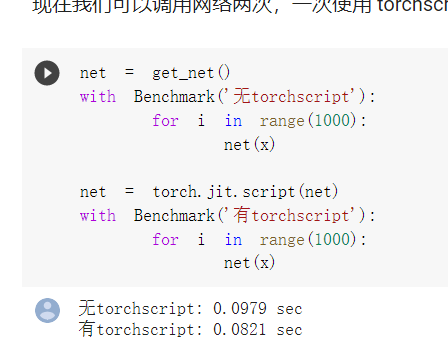# 编译器和解释器@应该是装饰器的意思，不是注释``````net = nn.Sequential(b1, b2, b3, b4, b5,
nn.Flatten(), nn.Linear(512, 10))
net = torch.jit.script(net)
``````

``````net = get_net()
with Benchmark('无torchscript'):
for i in range(100000): net(x)

net = torch.jit.script(net)
with Benchmark('有torchscript'):
for i in range(100000): net(x)